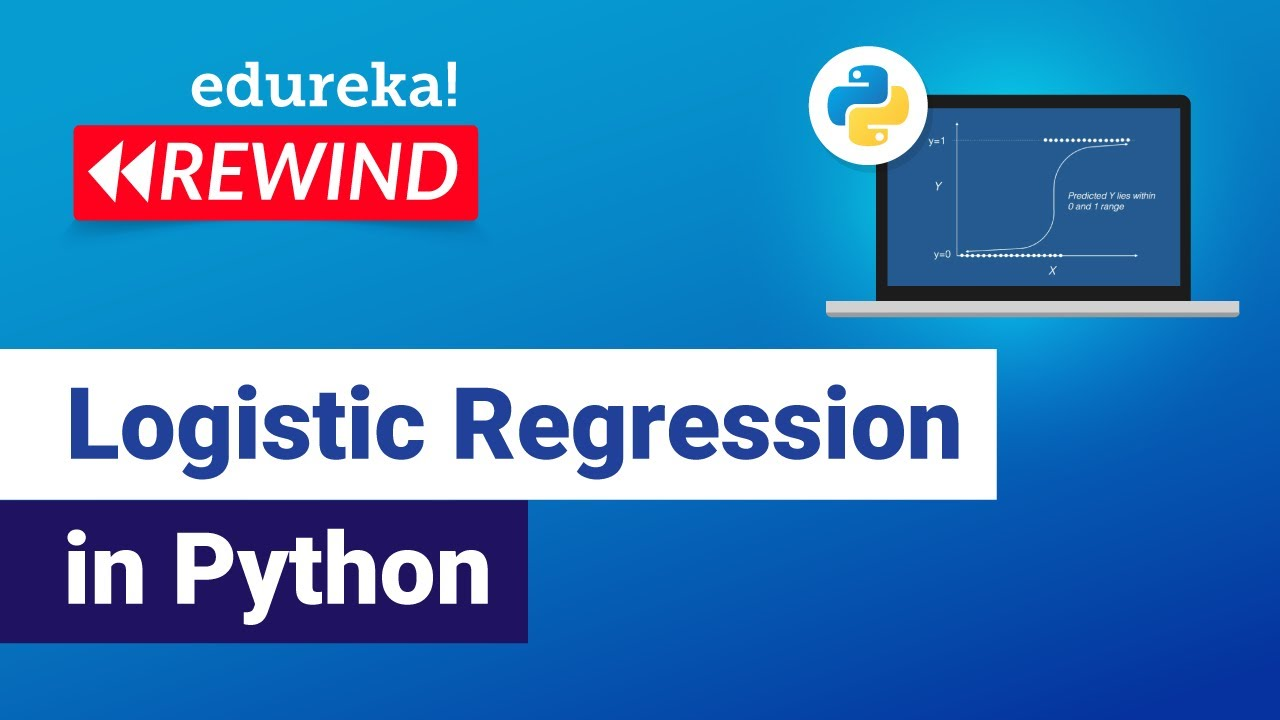# edureka pythonPython

#### Data Structures & Algorithms in Python Tutorial

This Edureka video on Regular Expressions in Python will help you understand the various fundamental concepts in Python and how they are used in Python programming along with examples.Python

#### Logistic Regression in Python

This Edureka Video on Logistic Regression in Python will give you basic understanding of Logistic Regression Machine Learning Algorithm with examples. Time stamps: 00:00 Introduction 00:31 Agenda 01:13 What is Regression 03:25 Logistic Regression- What and Why 04:10 Why not Linear Regression 05:18 Logistic Regression curve 06:57 Logistic Regression Equation 08:49 Linear vs Logistic Regression […]Python

#### Data Structures & Algorithms in Python

Edureka provides this free course on data structures and algorithms in Python. Enjoy.Uncategorized

#### Data Structures & Algorithms in Python

Edureka covers data structures and algorithms in Python in this livestreamed lecture.